# B.TECH 3rd sem Network Analysis paper 2016

UniversityVTU, Belgaum, Karnataka
CourseB.TECH
Semester3
SubjectNetwork Analysis
Year2016
Downloads2
Uploaded By###### Heman Sharma
100

 Question Marks 0 requests Using source transformation find current through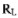in the circuit shown in the following figure.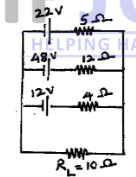This question has 0 answers so far. 6 0 requests Using mesh current method find current through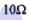resistor in the circuit shown in the following figure.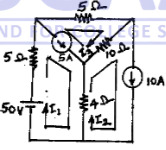. This question has 0 answers so far. 7 0 requests Find all the nodal voltages in the circuit shown in the following figure.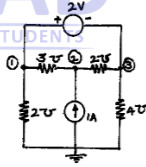This question has 0 answers so far. 7 0 requests With neat illustrations, distinguish betweeni) Oriented and Non-oriented graphs ii) Connected and un-connected graphs iii) Tree and co-tree. This question has 0 answers so far. 6 0 requests For the network shown in the following figure, draw the oriented graph. By selecting branches 4, 5 and 6 as twigs, write down tie-set schedule. Using this tie-set schedule, find all the branch currents and branch voltages.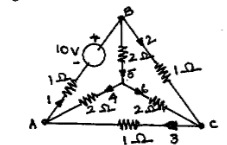This question has 0 answers so far. 14 0 requests State and illustrate superposition theorem. This question has 0 answers so far. 5 0 requests Using superposition theorem, find value of i in the circuit shown in the following figure.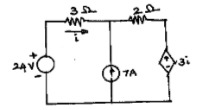. This question has 0 answers so far. 8 0 requests Show that the power delivered to load, when the load impedance consists of variable resistance and variable reactance is maximum when the load impedance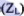is equal to complex conjugate of source impedance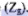. This question has 0 answers so far. 10 0 requests Obtain Thevenin's equivalent network of the circuit shown in the following figure and thereby find current through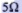resistor connected between terminals A and B.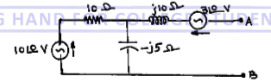This question has 0 answers so far. 10 0 requests Find the value of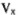in the circuit shown in the following figure. Verify it using Reciprocity theorem.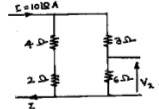This question has 0 answers so far. 7 0 requests With respect to series resonant circuit, define resonant frequency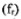and half power frequencies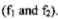. Also show that the resonant frequency is equal to the geometric mean of half power frequencies. This question has 0 answers so far. 10 0 requests A series circuit is energized by a constant voltage and constant frequency supply. Resonance takes place due to variation of inductance and the supply frequency is 300Hz. The capacitance in the circuit is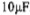. Determine the value of resistance in the circuit if the quality factor is 5. Also find the value of the inductance at half power frequencies. This question has 0 answers so far. 10 0 requests /In the circuit shown in the following figure, the switch K is changed from position A to B t=0. After having reached steady state in position A. Find i,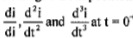at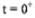.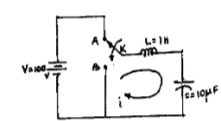This question has 0 answers so far. 10 0 requests In the circuit shown in the following figure, the switch K is opened at t = 0, Find i,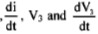at.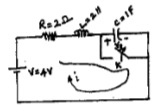This question has 0 answers so far. 10 0 requests Using convolution theorem find the inverse Laplace transform of following functions.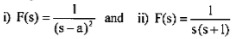. This question has 0 answers so far. 10 0 requests Obtain the Laplace transform of the triangular waveform shown in the following figure.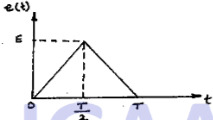This question has 0 answers so far. 10 0 requests Define h and T parameters of a two - port network. Also, derive the expressions for h parameters in terms of T parameters. This question has 0 answers so far. 10 0 requests Fina Y ana z parameters for the network shown in the following figure.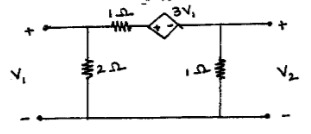This question has 0 answers so far. 10
Chat with us